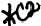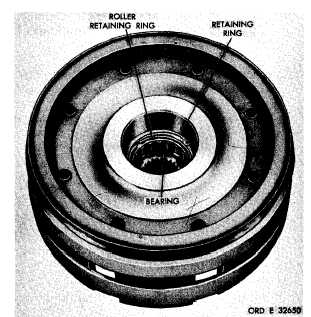Figure  231.  High-range  clutch  housing
Custom Search*C2 *C2 *C2 C H A P   5,   SEC  X X V I I H I G H     C L U T CH R E B U I LD PAR  0 - 2 01 .   I f    t h e    s l e e v e    w a s    r e m o v e d    f r o m    t he clutch   housing,   install   the   sleeve   3/4-inch   be- low   the   top   edge   of   the   housing   (fig.   230). .   I n s t a l l    a    h o o k - t y p e    s e a l    r i n g    o n    t he hub   of   the   high-range   clutch   housing   (fig.   230). .   I f    s e a l    r i n g    a n d    e x p a n d e r    w e r e    r e - moved   from   high-range   clutch   piston,   install new   replacements   (fig.   230). .   I n s t a l l    t h e    p i s t o n    i n    t h e    h i g h - r a n ge clutch   housing   (fig.   230). .   Install   13   clutch   piston   return   springs Figure  231.  High-range  clutch  housing 66   (fig.   375,   fold-out   4). 2 0 0 .    I N S P E C T I O N    A N D    R E P A IR .   I n s t a l l    t h e    h i g h - r a n g e    c l u t c h    p i s t on return   spring   retainer   (fig.   229). R e f e r    t o    p a r .    7 2    f o r    g e n e r a l    i n s p e c t i on and    repair    recommendations.    Repair    and    re- .   U s i n g    a    c o m p r e s s i n g    t o o l    ( f i g .    3 8 ) , b u i l d    p o i n t s    o f    m e a s u r e m e n t    f o r    f i t s ,    c l e a r- compress   piston   return   springs   and   install   the antes   and   wear   limits   are   indicated   by   small, lower   case   letters   in   fig.   375,   fold-out   4.   Re- fer   to   par.   238   for   wear   limits   information. 2 0 1 .    A S S E M B L Y    ( f i g .    3 7 5 ,    f o l d - o u t    4) .   If   the   bearing   was   removed   from   the high-range   clutch   housing,   install   a   new   re- placement.   Press   the   bearing   in   until   firmly s e a t e d    i n    t h e    h o u s i n g .    I n s t a l l    t h e    r e t a i n i ng ring   (fig.   231). .   I f    o n l y    b e a r i n g    r o l l e r s    w e r e    r e m o v ed from   the   clutch   housing   for   cleaning,   grease the   rollers   lightly   and   install   in   the   bearing r a c e .    I n s t a l l    t h e    r o l l e r    r e t a i n i n g    r i n g    ( f i g. 2 3 1 ) . retaining   ring   that   retains   the   retainer   (fig. 2 2 9 ). .   Install   the   bearing   on   the   hub   of   the h i g h - r a n g e    c l u t c h    h o u s i n g    ( f i g .    2 2 7 ) .    P r e ss the   bearing   inner   race   until   it   is   firmly   seated on   the   hub. .   Install   five   internal-   and   four   external- s p l i n e d    h i g h - r a n g e    c l u t c h    p l a t e s ,    b e g i n n i ng with   an   internal-splined   plate   (fig.   227). l.   Install    the    high-range    clutch    reaction plate   (fig.   227). .   Install   the   retaining   ring   that   retains high-range   clutch   reaction   plate   (fig.   227). 1 5 6Integrated Publishing, Inc. - A (SDVOSB) Service Disabled Veteran Owned Small Business